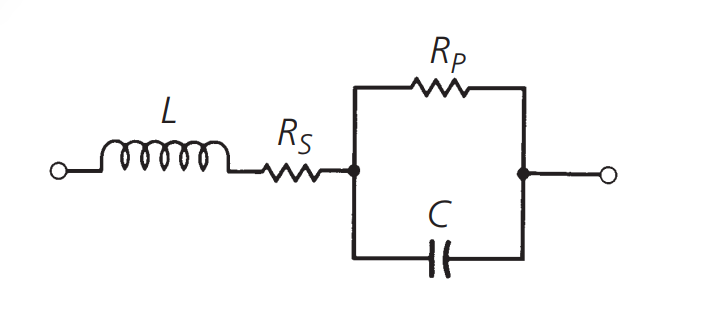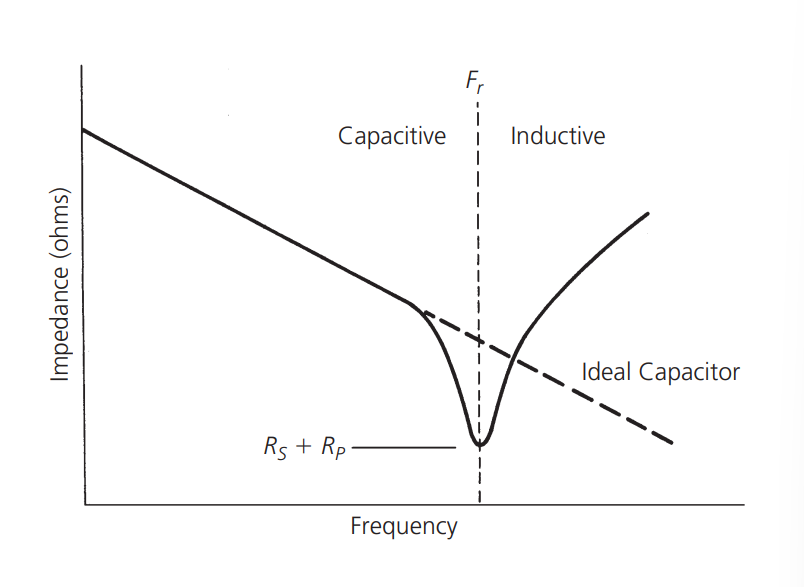# 射频 - 组件与系统 - 电容

## 平行板电容

$C=\frac{Q}{V}$

$C=\frac{0.2249\varepsilon A}{d\varepsilon_0} pF$

## 电容等效电路$PF=\cos \phi$

$ESR=\frac{PF}{\omega C}(10^6)$

$DF=\frac{ESR}{X_c}*100\%$（未完待续）

## 参考与致谢

• 《RF-Circuit-Design(second-edition)_Chris-Bowick》